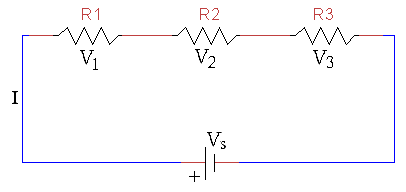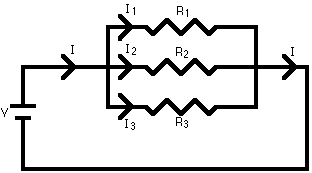### Concept of Series and Parallel Combination

Series Combination:
In a series circuit, all components are connected end to end to form a single path for current.
Resistor in series:
When resistors are connected in series, total voltage across all resistors is equal to the sum of the voltages across each resistor.V = V1 + V2 + V3
V ∝ R
Req = R1 + R2 + ……… +Rn

Parallel Combination:

In a parallel circuit, all components are connected in such a way such that it divides current in multiple paths.
Resistor in parallel:

When resistors are connected in parallel, the voltage across each resistor is the same, but current is different.
In a parallel resistor network, the circuit current can take more than one path as there are multiple paths for flow of current.
Hence, parallel circuits are also called current dividers.I = I1 + I2 + I3
I ∝ 1/R
1/Req = 1/R1 + 1/R2 +.......... +1/Rn

Unlike resistors, inductor and capacitor also perform series and parallel combinations.